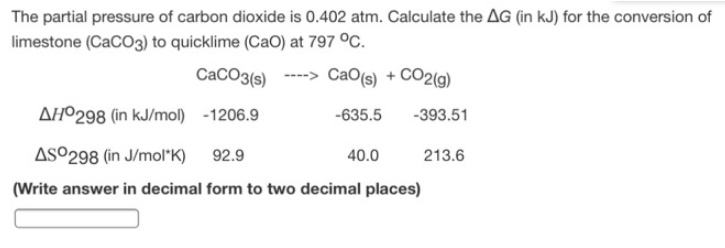# Problem: The partial pressure of carbon dioxide is 0.402 atm. Calculate the ΔG (in kJ) for the conversion limestone (CaCO3) to quicklime (CaO) at 797°C.                                        CaCO3 (s) → CaO (s) + CO2 (g) ΔH° 298 (in kJ/mol)       -1206.9         -635.5      -393.51 ΔS° 298 (in J/mol*K)       92.9              40.0         213.6 (Write answer in decimal form to two decimal places)

###### FREE Expert Solution
95% (298 ratings)###### Problem Details

The partial pressure of carbon dioxide is 0.402 atm. Calculate the ΔG (in kJ) for the conversion limestone (CaCO3) to quicklime (CaO) at 797°C.

CaCO3 (s) → CaO (s) + CO2 (g)

ΔH° 298 (in kJ/mol)       -1206.9         -635.5      -393.51

ΔS° 298 (in J/mol*K)       92.9              40.0         213.6

(Write answer in decimal form to two decimal places)